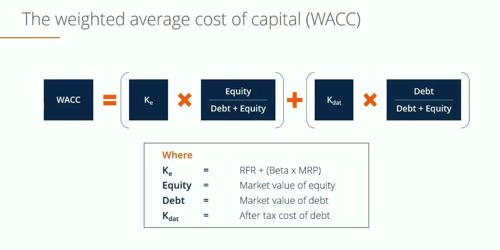Accounting

# Calculation Process of Weighted Average Cost of Capital (WACC)Weighted Average Cost of Capital (WACC) is calculated by multiplying the cost of each capital source (debt and equity) by its relevant weight and then adding the products together to determine the value. WACC is the average after-tax cost of a company’s various capital sources, including common stock, preferred stock, bonds, and any other long-term debt.

The WACC is the rate at which a company’s future cash flows need to be discounted to arrive at a present value for the business. Management typically uses this ratio to decide whether the company should use debt or equity to finance new purchases. This ratio is very comprehensive because it averages all sources of capital; including long-term debt, common stock, preferred stock, and bonds; to measure an average cost of borrowing funds.  It reflects the perceived riskiness of the cash flows.

Calculation Process of Weighted Average Cost of Capital (WACC)

The WACC formula is calculated by dividing the market value of the firm’s equity by the total market value of the company’s equity and debt multiplied by the cost of equity multiplied by the market value of the company’s debt by the total market value of the company’s equity and debt multiplied by the cost of debt times 1 minus the corporate income tax rate.

Step I: calculation of component or specific cost of capital:

• After-tax cost of debt (kdt)
• Cost of preference share (kp)
• Cost of equity share (ke)
• Cost of retained earnings( kr)

Step II: Calculation of proportion or weight of the source of capital:

• Proportion or weight of debt (Wd) = Amount of debt/Total Capital
• Weight of preference share (Wp) = Amount of preference share/Total capital
• Weight of equity share (We)= Amount of equity share/Total capital
• Weight of retained earnings (Wr)= Amount of retained earnings/ Total capital

Step III: Calculation of Weighted average cost of capital (WACC):

WACC = Wd x kdt+ Wp x kp+ We x ke + Wr x kr

The WACC calculation is pretty complex because there are so many different pieces involved, but there are really only two elements that are confusing: establishing the cost of equity and the cost of debt. After you have these two numbers figured out calculating WACC is a breeze.